# Chapter 10 Laboratory 2: Weather

## 10.1 Introduction

In recent lectures, we have discussed the earth’s energy balance and basics of precipitation formation and other meteorological phenomena. The main learning objectives of this lab are for you to become familiar with different types of instrumentation used in environmental science (specifically here, in climate and weather science), and more so, to learn how to manipulate and make calculations with large datasets using spreadsheet software. Weather is what we call the condition of the atmosphere at any particular time and place. Constantly changing, it is comprised of a number of variables, including:

• Humidity
• Precipitation
• Wind speed and direction
• Atmospheric pressure
• Air temperature
• Radiative or energy fluxes (such as insolation – incoming solar radiation)

Humidity can loosely be defined as the amount of water vapour in the air. In actuality, there are three main measurements of humidity: relative, absolute, and specific humidity, but relative humidity is the most often used. Relative humidity refers to the ratio of the amount of water vapour actually in the air to the maximum amount of water vapour required for saturation at that particular temperature and pressure—in other words, how close the air is to being saturated with vapour. Relative humidity can be used in conjunction with temperature to approximate the dew point—the atmospheric temperature at which a particular air mass will be saturated (100% relative humidity) with respect to water vapour—with the following formula developed by Mark G. Lawrence in 2005:

$\begin{equation} T_{dew} = T_{air} – \frac{(100-RH)}{5} \tag{10.1} \end{equation}$

where:

• $$T_{dew}$$ is the dew point in $$^\circ$$C
• $$T_{air}$$ is temperature in $$^\circ$$C
• $$RH$$ is relative humidity in %

If you know the dew point, you can calculate the actual vapour pressure (e) of the air mass using the formula:

$\begin{equation} e = 6.11 \times 10^\frac{(7.5\times T_{dew})}{(237.3+T_{dew})} \tag{10.2} \end{equation}$

where:

• $$e$$ is the vapour pressure in millibars (mb)
• $$T_{dew}$$ is the dew point in $$^\circ$$C

Once you have the vapour pressure, it is relatively easy to calculate the humidex (how hot the weather feels to the average person) with the following formula:

$\begin{equation} Humidex = T_{air} + 0.5555(e -10) \tag{10.3} \end{equation}$

where:

• $$T_{air}$$ is the air temperature in $$^\circ$$C
• $$e$$ is the vapour pressure in mb
Humidity can be measured in a number of ways, including instruments such as hygrometers, sling psychrometers, and electronic probes such as the HygroMer®, which are often used in a temperature-relative humidity electronic probe (Figure 10.1).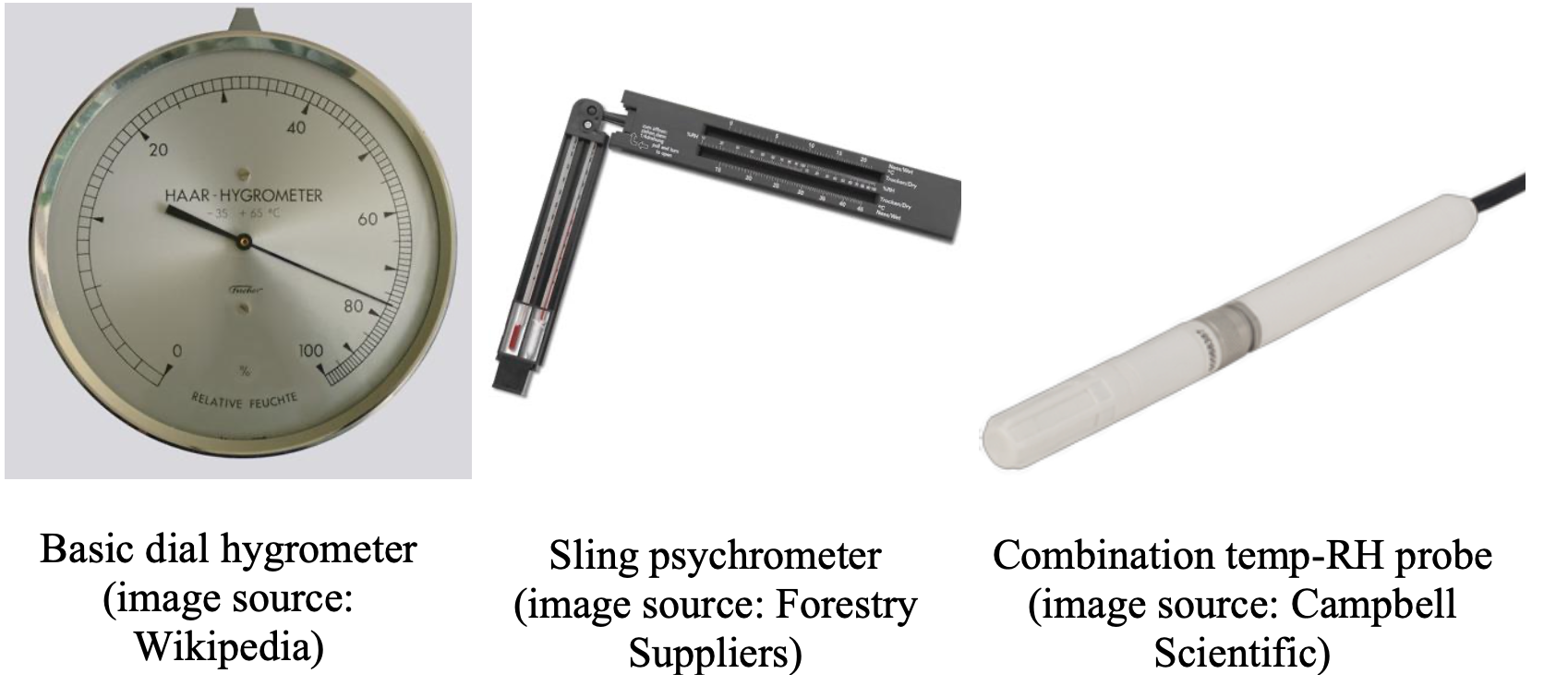Figure 10.1: Weather instruments that measure humidity.

Precipitation is the falling of either liquid or solid water particles from clouds to the Earth’s surface by the force of gravity. These liquid or solid particles are better known as rain, snow, or hail. Commonly, rain is measured using rain gauges and snow by a variety of techniques, including snow gauges (Figure 10.2).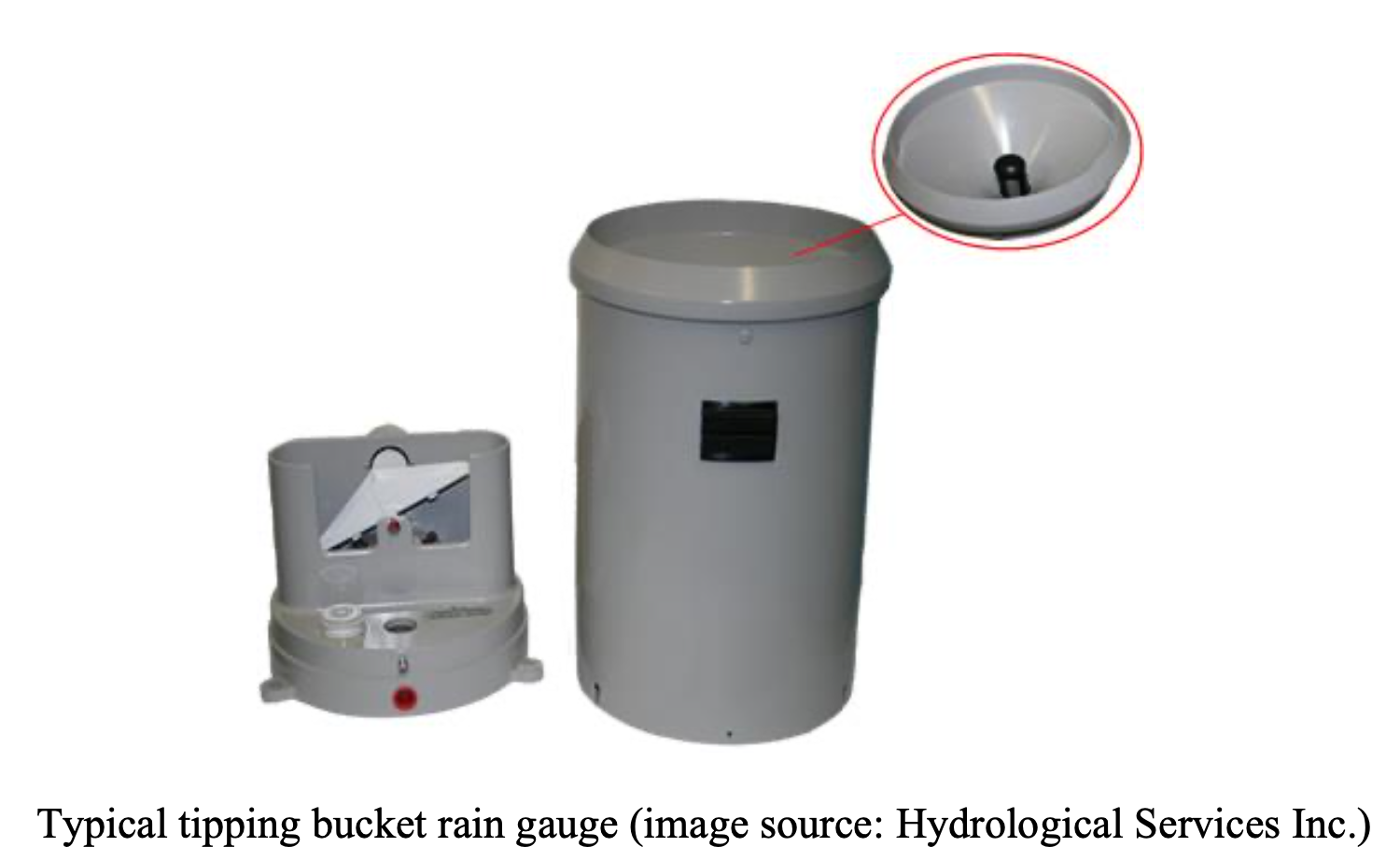Figure 10.2: A rain gauge.

Wind is the horizontal, bulk movement of air on the Earth’s surface. Winds are often discussed with regards to the direction (from which they are blowing) as well as their strength. Weather vanes (Figure 10.3) are used to measure wind direction, whereas anemometers are used to measure wind speed. Sometimes these two instruments can be combined into one instrument that can measure both wind direction and speed (as in the photo below).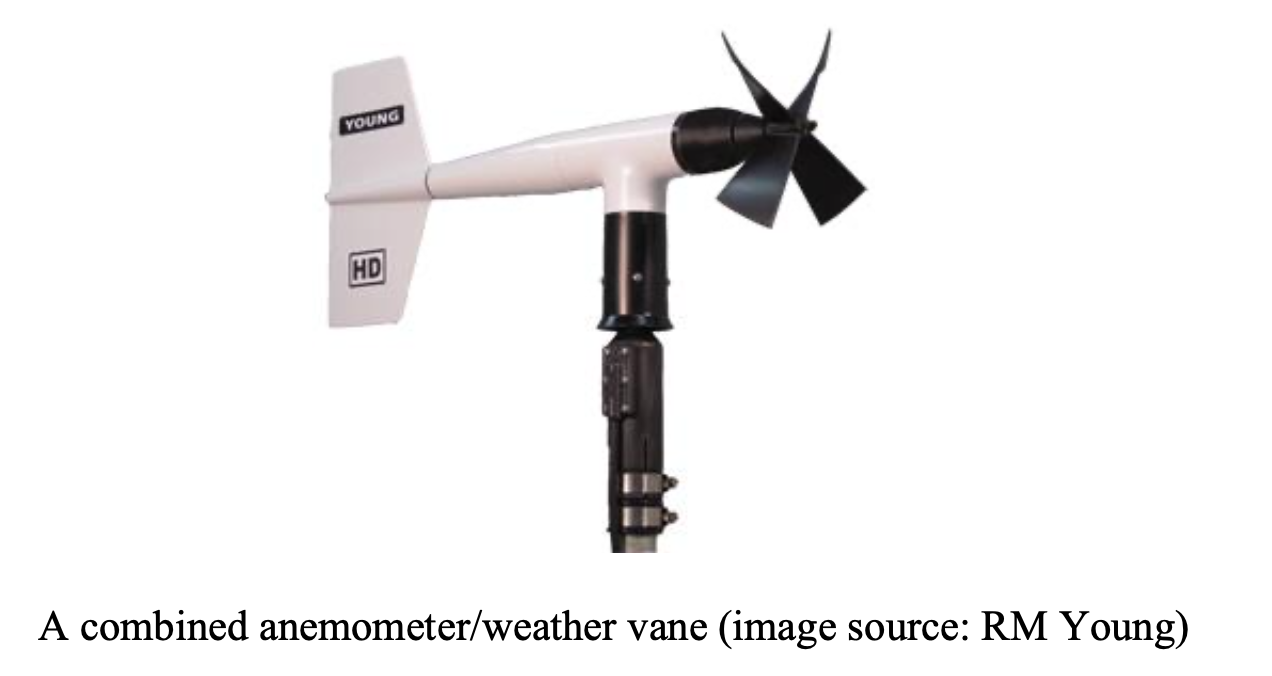Figure 10.3: A weather vane and anemometer.

Atmospheric or barometric pressure is caused by the pressure exerted by the weight of the air in the atmosphere of Earth. In other words, it is the amount of force exerted over an area of surface by the air above. The instrument used to measure atmospheric pressure is the barometer (Figure 10.4).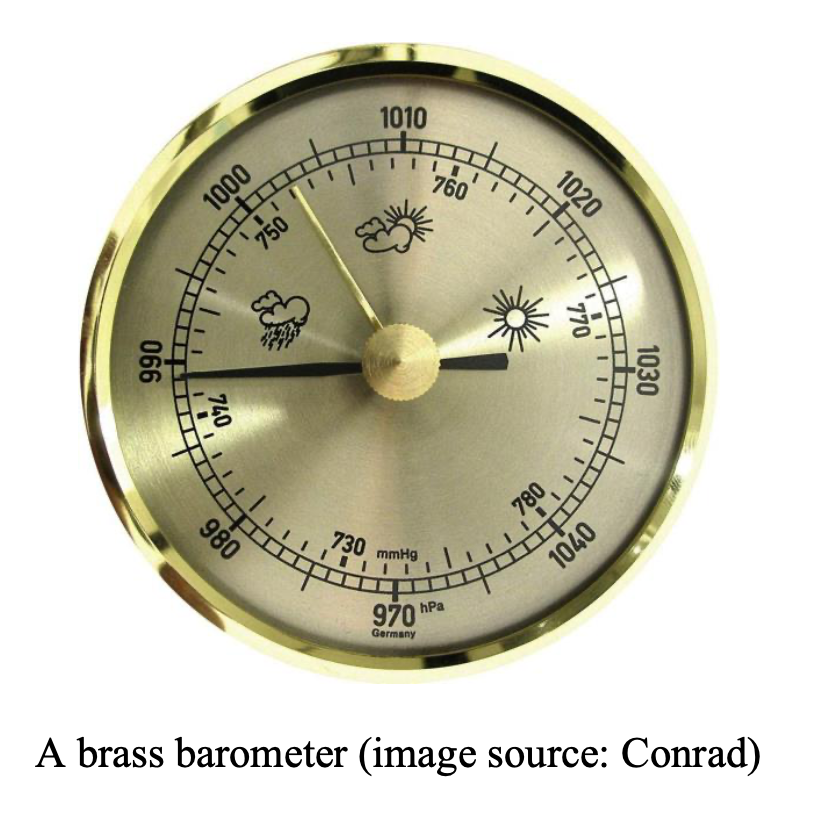Figure 10.4: A brass barometer.

Temperature is a measure of sensible energy (the energy that we can feel) and is one of the more intuitive meteorological measurements to most students. Thermometers (Figure 10.5) and a variety of electronic sensors, such as thermocouples and thermistors, are used to measure temperature.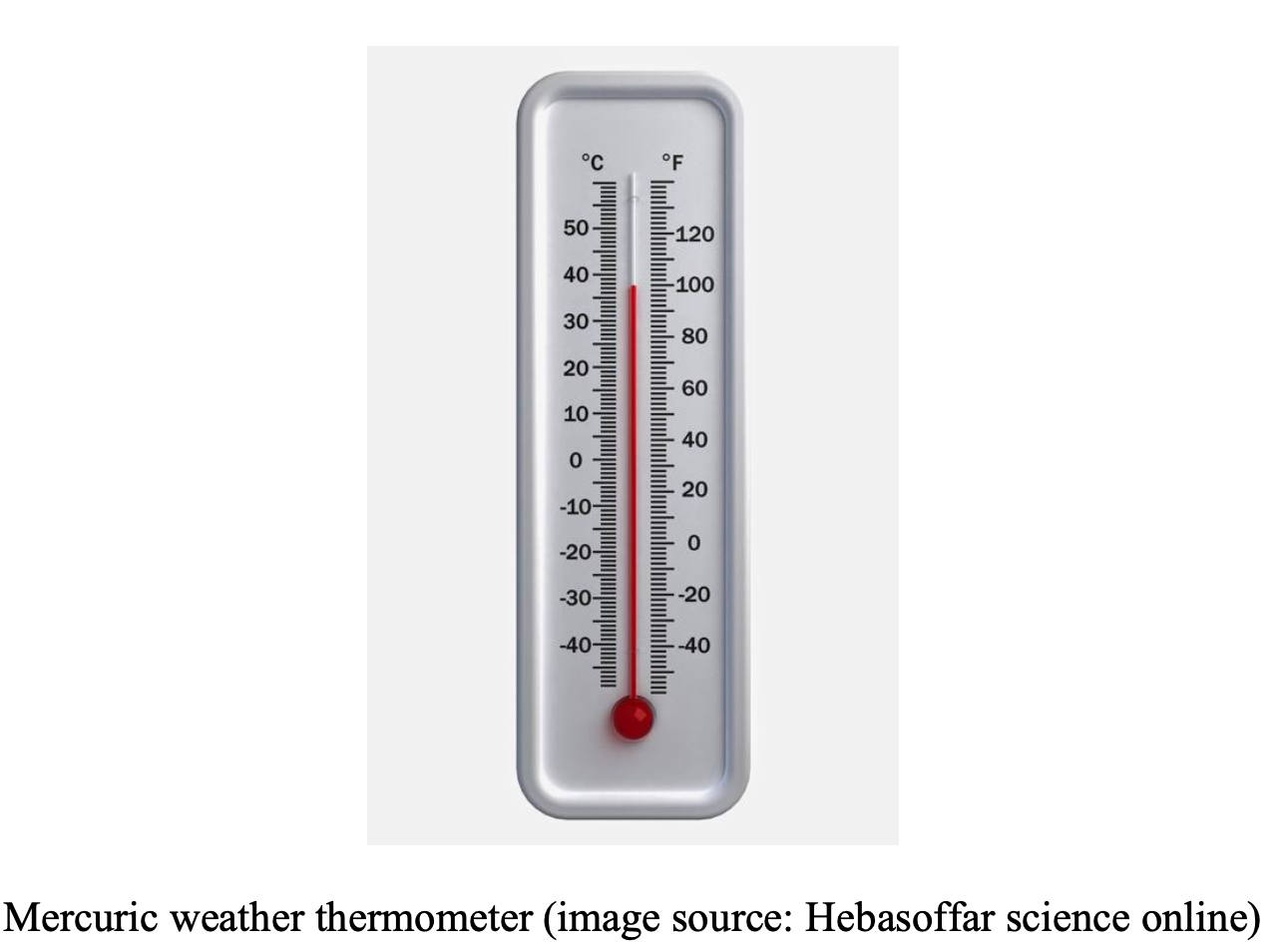Figure 10.5: A mercuric weather thermometer.

Solar irradiance, or the energy flux hitting the earth’s surface from the Sun, can be measured using a pyranometer. Instruments for measuring all of the above variables can be found both at the demonstration plot behind the Science Research Building and at the UTSC Weather Station on top of the Science Wing—it provides data for DPES and even has its own twitter account UTSC_weather, which posts autonomously four times a day with weather updates.Video 10.1: Virtual weather station tour: Click the image above to see the YouTube video created by Andrew Zajch and Tom Meulendyk!

## 10.2 Procedure for Laboratory 2

You should have a written account of what you have done in a given experiment. Making detailed notes during the lab will help you in answering the questions in the assignment.

1. Please meet in your regular lab room. It is very important that you arrive on time! Your TA will then lead the way to the UTSC weather station.

Please be on time! Your teaching assistant will not wait for you if you are late.

1. Familiarize yourself with how Microsoft Excel (or similar spreadsheet programs like Numbers for Mac) works and use spreadsheets to quickly make calculations in large datasets. Your teaching assistants will teach you the basics of using spreadsheets during this lab. You can find instructions on how to download Microsoft Office for free here: (Chapter 3.4)

2. Download the necessary files from Quercus and use a spreadsheet program to make large numbers of calculations and graphs, as instructed by your TA.

Make sure you have finished the following before you exit the laboratory:

• You have a written account of what you have observed during the lab
• You have cleaned up your work area

## 10.3 Assignment #2

Assignment 2 is 45 marks total – worth 10% of your final grade.
Please type and print your lab 2 assignment. Your teaching assistant will not collect lab assignments that are handwritten. Your lab 2 assignment is due at the beginning of lab 3.

Using the data provided from the UTSC weather station for the months of June and July of this year (available for download via Quercus), answer the following questions.

1. Provided are the UTSC weather station’s daily measurements over the months of June and July for the following meteorological variables:
• Average temperature in $$^\circ$$C (AirTC_AVG)
• Relative humidity in % saturation (RH)
• Barometric Pressure in kPa (BP_kPa_AVG)
• Total rainfall in mm (Rain_mm_TOT)
• Average windspeed in kilometers per hour (WS_kph_AVG)
• Wind direction in degrees ($$^\circ$$)
• Average solar irradiance in watts per m2 (SlrW_AVG)
• Total solar irradiance in kilojoules per m2 (SlrkJ_2_TOT)

Using this data, calculate the mean monthly values for anything that should be averaged for each of June and July or total monthly values for anything that should be summed using the appropriate Excel functions (see Appendix C). For submitting this question, submit a table (see Appendix F) for each of June and July data that clearly shows the sums or means (clearly labeled). (9 marks)

1. Calculate mean dew point for every day of both June and July (Refer to equation (10.1) to calculate the dewpoint). What is the mean dewpoint for each month of June and July? Calculate the vapour pressure for every day in both June and July (Refer to equation (10.2) to calculate the vapour pressure). Calculate the humidex for every day in both June and July (Refer to equation (10.3) to calculate the humidex). What is the mean humidex for each month of June and July? Organize your answer in a neat, clearly labeled table. (10 marks)

2. Using IF/THEN statements (see Appendix C), change the daily average wind direction to quadrants entitled “N”, “S”, “E”, and “W”. For “N”, use >315-45$$^\circ$$, for “S”, use >135-225$$^\circ$$, for “E”, use >45-135$$^\circ$$, and for “W”, use >225-315$$^\circ$$. Calculate the dominant wind direction for each month by calculating the “mode”. In your submitted answer, include the formulae (IF/THEN statements) you used to calculate each wind direction. (10 marks)

3. Make a scatterplot relating mean daily temperature to mean daily solar irradiance. Be mindful of which variables should be on the independent and dependent axes. Add a linear trendline to the data and include both an equation and R2 value for the trendline. Comment on the observed relationship in terms of its strength and why you would or would not expect a good relationship here. See Appendix (G) for more information on constructing a plot. (10 marks)

4. In less than 10 lines, explain how a vane anemometer works (do some research and use APA style referencing). What two meteorological variables does this instrument measure? See Appendix (H) for more information on APA referencing. (6 marks)